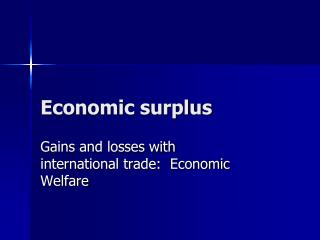Download PresentationEconomic surplusEconomic surplus - PowerPoint PPT Presentation

Download PresentationEconomic surplus
An Image/Link below is provided (as is) to download presentation

Download Policy: Content on the Website is provided to you AS IS for your information and personal use and may not be sold / licensed / shared on other websites without getting consent from its author. While downloading, if for some reason you are not able to download a presentation, the publisher may have deleted the file from their server.

- - - - - - - - - - - - - - - - - - - - - - - - - - - E N D - - - - - - - - - - - - - - - - - - - - - - - - - - -
Presentation Transcript

1. Economic surplus Gains and losses with international trade: Economic Welfare

2. Consumer surplus • Consumer surplus is the net gain to consumers being able to buy a product through a market • Consumer surplus is the difference between the highest price someone is willing to pay for a product and the actual market price that is paid, then summed over all units that are demanded and consumed

3. The highest price that someone is willing to pay for a unit of a product indicates the value that the buyer attaches to that unit • In order to measure consumer surplus, one has to have: • Market price, quantity demanded, and slope or shape of the demand curve

4. Consumer surplus can then be measured as the area below the demand curve and above the market-price line CONSUMER SURPLUS IS THE AREA GIVEN BY THE TRIANGE, C PRICE a a = the intercept of the inverse demand function, while P = market price, and Q = the quantity consumed at the price P C C P Price in the market DEMAND Q QUANTITY

5. AS DEMAND SHIFTS OUTWARD TO THE RIGHT, GIVEN THE SAME MARKET PRICE, THEN CONSUMER SURPLUS INCREASES CONSUMER SURPLUS IS THE AREA GIVEN BY THE TRIANGE, C a PRICE C ALSO RECALL THAT THE AREA OF A TRAINGLE IS ½ TIMES BASE TIMES HEIGHT C DEMAND P Price in the market Q QUANTITY SO CONSUMER SURPLUS IS THEREFORE, ½(a – p)Q

6. Now we impose an actual supply function and derive the price as the equilibrium price from the condition that demand = supply in the market A PERFECTLY COMPETITIVE MARKET IS ASSUMED HERE CONSUMER SURPLUS TRIANGLE PRICE NOW, WE HAVE ANOTHER SURPLUS CALLED PRODUCERS SURPLUS --- THE DIFFERENCE BETWEEN MARKET PRICE AND THE SUPPLY CURVE a SUPPLY E P DEMAND g Q QUANTITY g IS THE INTERCEPT OF THE INVERSE SUPPLY FUNCTION

7. LET’S USE AN ACTUAL DEMAND FUNCTION AND AN ACTUAL SUPPLY FUNCTION, BUT WITHOUT ANY INCOME EFFECT (IN DEMAND) AND WITHOUT ANY PRICE OF INPUTS (IN SUPPLY) • SUPPOSE THE DEMAND FUNCTION IS THEN GIVEN AS • QD = 18 – 1.2P, FOR QD = QUANTITY AND P = PRICE • LET THE SUPPLY FUNCTION BE GIVEN BY • QS = -2 + 0.6P, FOR QS = QUANTITY AND P = PRICE • WE NOW NEED THE EQUILIBRIUM PRICE AND QUANTITY IN THE MARKET • SET QD = QS, OR 18 – 1.2P = -2 + 0.6P • SOLVE FOR P BY REARRANGING AS 1.8P = 16, OR • P = 16/1.8 = 8.89 • THEN SUBSTITUTE P=8.89 INTO THE DEMAND FUNCTION TO GET Q = 18 – 1.2(8.89) = 7.33

8. SO EQUILIBRIUM PRICE IS 8.89 AND EQUILIBRIUM QUANTITY IN THE MARKET IS 7.33 • WE NOW WANT TO CALCULATE THE CONSUMER AND PRODUCER SURPLUS VALUES • INVERSE DEMAND FROM THE DEMAND FUNCTION Q = 18 – 1.2P IS GIVEN BY P = 18/1.2 – Q/1.2 • WHICH IS EQUAL TO P = 15 – 0.83Q • SO OUR INTERCEPT, a, IS NOW a = 15 • INVERSE SUPPLY FROM THE SUPPLY FUNCTION Q = -2 + 0.6P IS GIVEN BY P = 2/0.6 + Q/0.6 • WHICH IS EQUAL TO P = 3.33 + 1.67Q • SO OUR INVERSE SUPPLY INTERCEPT, g, IS NOW g = 3.33

9. NOW ON TO CONSUMER SURPLUS AND PRODUCER SURPLUS GIVEN EQUILIBRIUM PRICE IS P = 8.89; EQUILIBRIUM QUANTITY IS Q = 7.33; a = 15, AND g = 3.33 • CONSUMER SURPLUS = ½(15 – 8.89)(7.33) • WHICH IS APPROXIMATELY EQUAL TO 22.39 • PRODUCER SURPLUS = ½(8.89 – 3.33)(7.33) • WHICH IS APPROXIMATELY 20.38 CONSUMER SURPLUS = 22.39 PRICE, P a Actually Inverse supply Equilibrium price, P Actually inverse demand PRODUCER SURPLUS = 20.38 g Equilibrium quantity Q QUANTITY, Q

10. THE AREA UNDER THE SUPPLY CURVE AND UP TO A QUANTITY SUPPLIED OF Q IS THE PAYMENT TO VARIABLE INPUTS (VARIABLE COSTS) --- SO THE PRODUCER RECEIVES A SURPLUS OVER VARIABLE COSTS --- PRODUCERS SURPLUS CONSUMER SURPLUS = AREA aEP PRICE a SUPPLY P IS EQUILIBRIUM PRICE E P SUPPLY = DEMAND AT POINT E DEMAND g PRODUCERS SURPLUS = AREA gPE Q QUANTITY Q IS EQUILIBRIUM QUANTITY